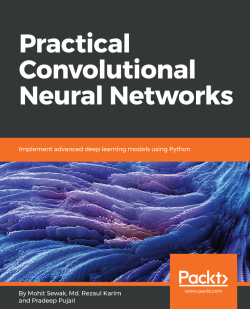# Practical Convolutional Neural Networks3.8 (10 reviews total)
By Mohit Sewak , Md. Rezaul Karim , Pradeep Pujari
• \$28.99 eBook
• \$36.99 Print + eBook
• \$12.99 eBook + Subscription
What do you get with a Packt Subscription?

• Constantly updated with 100+ new titles each month
• Breadth and depth in over 1,000+ technologies

Convolutional Neural Network (CNN) is revolutionizing several application domains such as visual recognition systems, self-driving cars, medical discoveries, innovative eCommerce and more.You will learn to create innovative solutions around image and video analytics to solve complex machine learning and computer vision related problems and implement real-life CNN models.

This book starts with an overview of deep neural networkswith the example of image classification and walks you through building your first CNN for human face detector. We will learn to use concepts like transfer learning with CNN, and Auto-Encoders to build very powerful models, even when not much of supervised training data of labeled images is available.

Later we build upon the learning achieved to build advanced vision related algorithms for object detection, instance segmentation, generative adversarial networks, image captioning, attention mechanisms for vision, and recurrent models for vision.

By the end of this book, you should be ready to implement advanced, effective and efficient CNN models at your professional project or personal initiatives by working on complex image and video datasets.

Publication date:
February 2018
Publisher
Packt
Pages
218
ISBN
9781788392303

## Chapter 1. Deep Neural Networks – Overview

In the past few years, we have seen remarkable progress in the field of AI (deep learning). Today, deep learning is the cornerstone of many advanced technological applications, from self-driving cars to generating art and music. Scientists aim to help computers to not only understand speech but also speak in natural languages. Deep learning is a kind of machine learning method that is based on learning data representation as opposed to task-specific algorithms. Deep learning enables the computer to build complex concepts from simpler and smaller concepts. For example, a deep learning system recognizes the image of a person by combining lower label edges and corners and combines them into parts of the body in a hierarchical way. The day is not so far away when deep learning will be extended to applications that enable machines to think on their own.

In this chapter, we will cover the following topics:

• Building blocks of a neural network
• Introduction to TensorFlow
• Introduction to Keras
• Backpropagation

## Building blocks of a neural network

A neural network is made up of many artificial neurons. Is it a representation of the brain or is it a mathematical representation of some knowledge? Here, we will simply try to understand how a neural network is used in practice. A convolutional neural network (CNN) is a very special kind of multi-layer neural network. CNN is designed to recognize visual patterns directly from images with minimal processing. A graphical representation of this network is produced in the following image. The field of neural networks was originally inspired by the goal of modeling biological neural systems, but since then it has branched in different directions and has become a matter of engineering and attaining good results in machine learning tasks.

An artificial neuron is a function that takes an input and produces an output. The number of neurons that are used depends on the task at hand. It could be as low as two or as many as several thousands. There are numerous ways of connecting artificial neurons together to create a CNN. One such topology that is commonly used is known as a feed-forward network:Each neuron receives inputs from other neurons. The effect of each input line on the neuron is controlled by the weight. The weight can be positive or negative. The entire neural network learns to perform useful computations for recognizing objects by understanding the language. Now, we can connect those neurons into a network known as a feed-forward network. This means that the neurons in each layer feed their output forward to the next layer until we get a final output. This can be written as follows:The preceding forward-propagating neuron can be implemented as follows:

```import numpy as np
import math

class Neuron(object):
def __init__(self):
self.weights = np.array([1.0, 2.0])
self.bias = 0.0
def forward(self, inputs):
""" Assuming that inputs and weights are 1-D numpy arrays and the bias is a number """
a_cell_sum = np.sum(inputs * self.weights) + self.bias
result = 1.0 / (1.0 + math.exp(-a_cell_sum)) # This is the sigmoid activation function
return result
neuron = Neuron()
output = neuron.forward(np.array([1,1]))
print(output)```

## Introduction to TensorFlow

TensorFlow is based on graph-based computation. Consider the following math expression, for example:

c=(a+b)d = b + 5,

e = c * d

In TensorFlow, this is represented as a computational graph, as shown here. This is powerful because computations are done in parallel: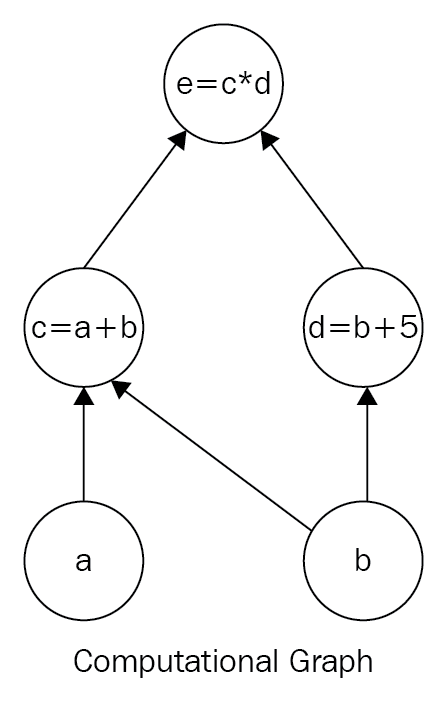### Installing TensorFlow

There are two easy ways to install TensorFlow:

• Using a virtual environment (recommended and described here)
• With a Docker image

#### For macOS X/Linux variants

The following code snippet creates a Python virtual environment and installs TensorFlow in that environment. You should have Anaconda installed before you run this code:

```#Creates a virtual environment named "tensorflow_env" assuming that python 3.7 version is already installed.
conda create -n tensorflow_env python=3.7
#Activate points to the environment named "tensorflow" source activate tensorflow_env
conda install pandas matplotlib jupyter notebook scipy scikit-learn
#installs latest tensorflow version into environment tensorflow_env
pip3 install tensorflow ```

Please check out the latest updates on the official TensorFlow page, https://www.tensorflow.org/install/.

Try running the following code in your Python console to validate your installation. The console should print `Hello World!` if TensorFlow is installed and working:

```import tensorflow as tf

#Creating TensorFlow object
hello_constant = tf.constant('Hello World!', name = 'hello_constant')
#Creating a session object for execution of the computational graph
with tf.Session() as sess:
#Implementing the tf.constant operation in the session
output = sess.run(hello_constant)
print(output)```

#### TensorFlow basics

In TensorFlow, data isn't stored as integers, floats, strings, or other primitives. These values are encapsulated in an object called a tensor. It consists of a set of primitive values shaped into an array of any number of dimensions. The number of dimensions in a tensor is called its rank. In the preceding example, `hello_constant` is a constant string tensor with rank zero. A few more examples of constant tensors are as follows:

```# A is an int32 tensor with rank = 0
A = tf.constant(123)
# B is an int32 tensor with dimension of 1 ( rank = 1 )
B = tf.constant([123,456,789])
# C is an int32 2- dimensional tensor
C = tf.constant([ [123,456,789], [222,333,444] ])```

TensorFlow's core program is based on the idea of a computational graph. A computational graph is a directed graph consisting of the following two parts:

• Building a computational graph
• Running a computational graph

A computational graph executes within a session. A TensorFlow session is a runtime environment for the computational graph. It allocates the CPU or GPU and maintains the state of the TensorFlow runtime. The following code creates a session instance named `sess` using `tf.Session`. Then the `sess.run()` function evaluates the tensor and returns the results stored in the `output` variable. It finally prints as `Hello World!`:

```with tf.Session() as sess:
# Run the tf.constant operation in the session
output = sess.run(hello_constant)
print(output)```

Using TensorBoard, we can visualize the graph. To run TensorBoard, use the following command:

`tensorboard --logdir=path/to/log-directory`

Let's create a piece of simple addition code as follows. Create a constant integer `x` with value `5`, set the value of a new variable `y` after adding `5` to it, and print it:

```constant_x = tf.constant(5, name='constant_x')
variable_y = tf.Variable(x + 5, name='variable_y')
print (variable_y)```

The difference is that `variable_y` isn't given the current value of `x + 5` as it should in Python code. Instead, it is an equation; that means, when `variable_y` is computed, take the value of `x` at that point in time and add `5` to it. The computation of the value of `variable_y` is never actually performed in the preceding code. This piece of code actually belongs to the computational graph building section of a typical TensorFlow program. After running this, you'll get something like `<tensorflow.python.ops.variables.Variable object at 0x7f074bfd9ef0>` and not the actual value of `variable_y` as `10`. To fix this, we have to execute the code section of the computational graph, which looks like this:

```#initialize all variables
init = tf.global_variables_initializer()
# All variables are now initialized

with tf.Session() as sess:
sess.run(init)
print(sess.run(variable_y))```

Here is the execution of some basic math functions, such as addition, subtraction, multiplication, and division with tensors. For more math functions, please refer to the documentation:

For TensorFlow math functions, go to https://www.tensorflow.org/versions/r0.12/api_docs/python/math_ops/basic_math_functions.

#### Basic math with TensorFlow

The `tf.add()` function takes two numbers, two tensors, or one of each, and it returns their sum as a tensor:

```Addition
x = tf.add(1, 2, name=None) # 3```

Here's an example with subtraction and multiplication:

```x = tf.subtract(1, 2,name=None) # -1
y = tf.multiply(2, 5,name=None) # 10
```

What if we want to use a non-constant? How to feed an input dataset to TensorFlow? For this, TensorFlow provides an API, `tf.placeholder()`, and uses `feed_dict`.

A `placeholder` is a variable that data is assigned to later in the `tf.session.run()` function. With the help of this, our operations can be created and we can build our computational graph without needing the data. Afterwards, this data is fed into the graph through these placeholders with the help of the `feed_dict` parameter in `tf.session.run()` to set the `placeholder` tensor. In the following example, the tensor `x` is set to the string `Hello World` before the session runs:

```x = tf.placeholder(tf.string)

with tf.Session() as sess:
output = sess.run(x, feed_dict={x: 'Hello World'})```

It's also possible to set more than one tensor using `feed_dict`, as follows:

```x = tf.placeholder(tf.string)
y = tf.placeholder(tf.int32, None)
z = tf.placeholder(tf.float32, None)

with tf.Session() as sess:
output = sess.run(x, feed_dict={x: 'Welcome to CNN', y: 123, z: 123.45}) ```

Placeholders can also allow storage of arrays with the help of multiple dimensions. Please see the following example:

```import tensorflow as tf

x = tf.placeholder("float", [None, 3])
y = x * 2

with tf.Session() as session:
input_data = [[1, 2, 3],
[4, 5, 6],]
result = session.run(y, feed_dict={x: input_data})
print(result)```

### Note

This will throw an error as `ValueError: invalid literal for...` in cases where the data passed to the `feed_dict` parameter doesn't match the tensor type and can't be cast into the tensor type.

The `tf.truncated_normal()` function returns a tensor with random values from a normal distribution. This is mostly used for weight initialization in a network:

```n_features = 5
n_labels = 2
weights = tf.truncated_normal((n_features, n_labels))
with tf.Session() as sess:
print(sess.run(weights))```

#### Softmax in TensorFlow

The softmax function converts its inputs, known as logit or logit scores, to be between 0 and 1, and also normalizes the outputs so that they all sum up to 1. In other words, the softmax function turns your logits into probabilities. Mathematically, the softmax function is defined as follows:In TensorFlow, the softmax function is implemented. It takes logits and returns softmax activations that have the same type and shape as input logits, as shown in the following image: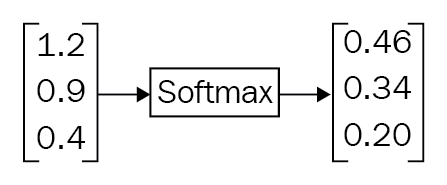The following code is used to implement this:

```logit_data = [2.0, 1.0, 0.1]
logits = tf.placeholder(tf.float32)
softmax = tf.nn.softmax(logits)

with tf.Session() as sess:
output = sess.run(softmax, feed_dict={logits: logit_data})
print( output )```

The way we represent labels mathematically is often called one-hot encoding. Each label is represented by a vector that has 1.0 for the correct label and 0.0 for everything else. This works well for most problem cases. However, when the problem has millions of labels, one-hot encoding is not efficient, since most of the vector elements are zeros. We measure the similarity distance between two probability vectors, known as cross-entropy and denoted by D.

### Note

Cross-entropy is not symmetric. That means: D(S,L) != D(L,S)

In machine learning, we define what it means for a model to be bad usually by a mathematical function. This function is called loss, cost, or objective function. One very common function used to determine the loss of a model is called the cross-entropy loss. This concept came from information theory (for more on this, please refer to Visual Information Theory at https://colah.github.io/posts/2015-09-Visual-Information/). Intuitively, the loss will be high if the model does a poor job of classifying on the training data, and it will be lowotherwise, as shown here: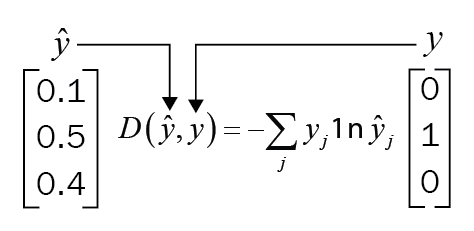Cross-entropy loss function

In TensorFlow, we can write a cross-entropy function using `tf.reduce_sum()`; it takes an array of numbers and returns its sum as a tensor (see the following code block):

```x = tf.constant([[1,1,1], [1,1,1]])
with tf.Session() as sess:
print(sess.run(tf.reduce_sum([1,2,3]))) #returns 6
print(sess.run(tf.reduce_sum(x,0))) #sum along x axis, prints [2,2,2]```

But in practice, while computing the softmax function, intermediate terms may be very large due to the exponentials. So, dividing large numbers can be numerically unstable. We should use TensorFlow's provided softmax and cross-entropy loss API. The following code snippet manually calculates cross-entropy loss and also prints the same using the TensorFlow API:

```import tensorflow as tf

softmax_data = [0.1,0.5,0.4]
onehot_data = [0.0,1.0,0.0]

softmax = tf.placeholder(tf.float32)
onehot_encoding = tf.placeholder(tf.float32)

cross_entropy = - tf.reduce_sum(tf.multiply(onehot_encoding,tf.log(softmax)))

cross_entropy_loss = tf.nn.softmax_cross_entropy_with_logits(logits=tf.log(softmax), labels=onehot_encoding)

with tf.Session() as session:
print(session.run(cross_entropy,feed_dict={softmax:softmax_data, onehot_encoding:onehot_data} ))
print(session.run(cross_entropy_loss,feed_dict={softmax:softmax_data, onehot_encoding:onehot_data} ))

```

## Introduction to the MNIST dataset

Here we use MNIST (Modified National Institute of Standards and Technology), which consists of images of handwritten numbers and their labels. Since its release in 1999, this classic dataset is used for benchmarking classification algorithms.

The data files `train.csv` and `test.csv` consist of hand-drawn digits, from 0 through 9 in the form of gray-scale images. A digital image is a mathematical function of the form f(x,y)=pixel value. The images are two dimensional.

We can perform any mathematical function on the image. By computing the gradient on the image, we can measure how fast pixel values are changing and the direction in which they are changing. For image recognition, we convert the image into grayscale for simplicity and have one color channel. RGB representation of an image consists of three color channels, RED, BLUE, and GREEN. In the RGB color scheme, an image is a stack of three images RED, BLUE, and GREEN. In a grayscale color scheme, color is not important. Color images are computationally harder to analyze because they take more space in memory. Intensity, which is a measure of the lightness and darkness of an image, is very useful for recognizing objects. In some applications, for example, detecting lane lines in a self-driving car application, color is important because it has to distinguish yellow lanes and white lanes. A grayscale image does not provide enough information to distinguish between white and yellow lane lines.

Any grayscale image is interpreted by the computer as a matrix with one entry for each image pixel. Each image is 28 x 28 pixels in height and width, to give a sum of 784 pixels. Each pixel has a single pixel-value associated with it. This value indicates the lightness or darkness of that particular pixel. This pixel-value is an integer ranging from 0 to 255, where a value of zero means darkest and 255 is the whitest, and a gray pixel is between 0 and 255.

### The simplest artificial neural network

The following image represents a simple two-layer neural network: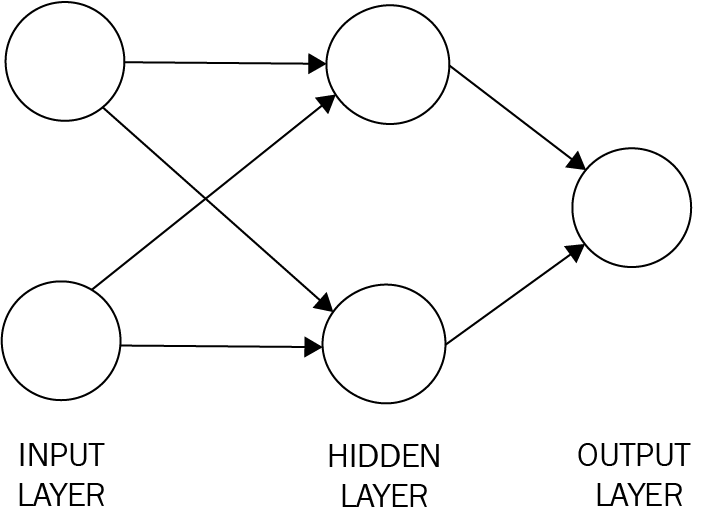Simple two-layer neural net

The first layer is the input layer and the last layer is the output layer. The middle layer is the hidden layer. If there is more than one hidden layer, then such a network is a deep neural network.

The input and output of each neuron in the hidden layer is connected to each neuron in the next layer. There can be any number of neurons in each layer depending on the problem. Let us consider an example. The simple example which you may already know is the popular hand written digit recognition that detects a number, say 5. This network will accept an image of 5 and will output 1 or 0. A 1 is to indicate the image in fact is a 5 and 0 otherwise. Once the network is created, it has to be trained. We can initialize with random weights and then feed input samples known as the training dataset. For each input sample, we check the output, compute the error rate and then adjust the weights so that whenever it sees 5 it outputs 1 and for everything else it outputs a zero. This type of training is called supervised learning and the method of adjusting the weights is called backpropagation. When constructing artificial neural network models, one of the primary considerations is how to choose activation functions for hidden and output layers. The three most commonly used activation functions are the sigmoid function, hyperbolic tangent function, and Rectified Linear Unit (ReLU). The beauty of the sigmoid function is that its derivative is evaluated at z and is simply z multiplied by 1-minus z. That means:

dy/dx =σ(x)(1−σ(x))

This helps us to efficiently calculate gradients used in neural networks in a convenient manner. If the feed-forward activations of the logistic function for a given layer is kept in memory, the gradients for that particular layer can be evaluated with the help of simple multiplication and subtraction rather than implementing and re-evaluating the sigmoid function, since it requires extra exponentiation. The following image shows us the ReLU activation function, which is zero when x < 0 and then linear with slope 1 when x > 0: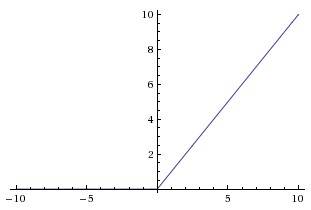The ReLU is a nonlinear function that computes the function f(x)=max(0x). That means a ReLU function is 0 for negative inputs and x for all inputs x >0. This means that the activation is thresholded at zero (see the preceding image on the left). TensorFlow implements the ReLU function in `tf.nn.relu()`:Backpropagation, an abbreviation for "backward propagation of errors", is a common method of training artificial neural networks used in conjunction with an optimization method such as gradient descent. The method calculates the gradient of a loss function with respect to all the weights in the network. The optimization method is fed with the gradient and uses it to get the weights updated to reduce the loss function.

#### Building a single-layer neural network with TensorFlow

Let us build a single-layer neural net with TensorFlow step by step. In this example, we'll be using the MNIST dataset. This dataset is a set of 28 x 28 pixel grayscale images of hand written digits. This dataset consists of 55,000 training data, 10,000 test data, and 5,000 validation data. Every MNIST data point has two parts: an image of a handwritten digit and a corresponding label. The following code block loads data. `one_hot=True` means that the labels are one-hot encoded vectors instead of actual digits of the label. For example, if the label is `2`, you will see [0,0,1,0,0,0,0,0,0,0]. This allows us to directly use it in the output layer of the network:

```from tensorflow.examples.tutorials.mnist import input_data

Setting up placeholders and variables is done as follows:

`# All the pixels in the image (28 * 28 = 784)features_count=784# there are 10 digits i.e labelslabels_count=10batch_size=128epochs=10learning_rate=0.5features=tf.placeholder(tf.float32,[None,features_count])labels=tf.placeholder(tf.float32,[None,labels_count])#Set the weights and biases tensorsweights=tf.Variable(tf.truncated_normal((features_count,labels_count)))biases=tf.Variable(tf.zeros(labels_count),name='biases')`

Let's set up the optimizer in TensorFlow:

```loss,

Before we begin training, let's set up the variable initialization operation and an operation to measure the accuracy of our predictions, as follows:

`# Linear Function WX + blogits=tf.add(tf.matmul(features,weights),biases)prediction=tf.nn.softmax(logits)# Cross entropycross_entropy=-tf.reduce_sum(labels*tf.log(prediction),reduction_indices=1)# Training lossloss=tf.reduce_mean(cross_entropy)# Initializing all variablesinit=tf.global_variables_initializer()# Determining if the predictions are accurateis_correct_prediction=tf.equal(tf.argmax(prediction,1),tf.argmax(labels,1))# Calculating prediction accuracyaccuracy=tf.reduce_mean(tf.cast(is_correct_prediction,tf.float32))`

Now we can begin training the model, as shown in the following code snippet:

`#Beginning the sessionwithtf.Session()assess:# initializing all the variablessess.run(init)total_batch=int(len(mnist.train.labels)/batch_size)forepochinrange(epochs):avg_cost=0foriinrange(total_batch):batch_x,batch_y=mnist.train.next_batch(batch_size=batch_size)_,c=sess.run([optimizer,loss],feed_dict={features:batch_x,labels:batch_y})avg_cost+=c/total_batchprint("Epoch:",(epoch+1),"cost =","{:.3f}".format(avg_cost))print(sess.run(accuracy,feed_dict={features:mnist.test.images,labels:mnist.test.labels}))`

## Keras deep learning library overview

Keras is a high-level deep neural networks API in Python that runs on top of TensorFlow, CNTK, or Theano.

Here are some core concepts you need to know for working with Keras. TensorFlow is a deep learning library for numerical computation and machine intelligence. It is open source and uses data flow graphs for numerical computation. Mathematical operations are represented by nodes and multidimensional data arrays; that is, tensors are represented by graph edges. This framework is extremely technical and hence it is probably difficult for data analysts. Keras makes deep neural network coding simple. It also runs seamlessly on CPU and GPU machines.

A model is the core data structure of Keras. The sequential model, which consists of a linear stack of layers, is the simplest type of model. It provides common functions, such as `fit()`, `evaluate()`, and `compile()`.

You can create a sequential model with the help of the following lines of code:

```from keras.models import Sequential

#Creating the Sequentialmodelmodel = Sequential()```

### Layers in the Keras model

A Keras layer is just like a neural network layer. There are fully connected layers, max pool layers, and activation layers. A layer can be added to the model using the model's `add()` function. For example, a simple model can be represented by the following:

```from keras.models import Sequential
from keras.layers.core import Dense, Activation, Flatten

#Creating the Sequentialmodelmodel = Sequential()

#Layer 1 - Adding a flatten layer

#Layer 2 - Adding a fully connected layer

#Layer 3 - Adding a ReLU activation layer

#Layer 4- Adding a fully connected layer

#Layer 5 - Adding an ReLU activation layer

Keras will automatically infer the shape of all layers after the first layer. This means you only have to set the input dimensions for the first layer. The first layer from the preceding code snippet, `model.add(Flatten(input_shape=(32, 32, 3)))`, sets the input dimension to (32, 32, 3) and the output dimension to (3072=32 x 32 x 3). The second layer takes in the output of the first layer and sets the output dimensions to (100). This chain of passing the output to the next layer continues until the last layer, which is the output of the model.

## Handwritten number recognition with Keras and MNIST

A typical neural network for a digit recognizer may have 784 input pixels connected to 1,000 neurons in the hidden layer, which in turn connects to 10 output targets — one for each digit. Each layer is fully connected to the layer above. A graphical representation of this network is shown as follows, where `x` are the inputs, `h` are the hidden neurons, and `y` are the output class variables: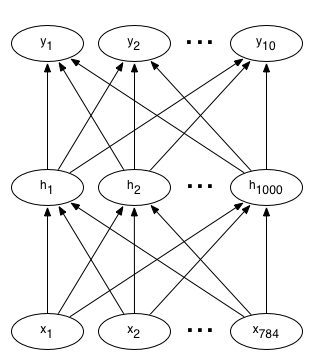In this notebook, we will build a neural network that will recognize handwritten numbers from 0-9.

The type of neural network that we are building is used in a number of real-world applications, such as recognizing phone numbers and sorting postal mail by address. To build this network, we will use the MNIST dataset.

We will begin as shown in the following code by importing all the required modules, after which the data will be loaded, and then finally building the network:

`# Import Numpy, keras and MNIST dataimportnumpyasnpimportmatplotlib.pyplotaspltfromkeras.datasetsimportmnistfromkeras.modelsimportSequentialfromkeras.layers.coreimportDense,Dropout,Activationfromkeras.utilsimportnp_utils`

### Retrieving training and test data

The MNIST dataset already comprises both training and test data. There are 60,000 data points of training data and 10,000 points of test data. If you do not have the data file locally at the `'~/.keras/datasets/' +` path, it can be downloaded at this location.

Each MNIST data point has:

• An image of a handwritten digit
• A corresponding label that is a number from 0-9 to help identify the image

The images will be called, and will be the input to our neural network, X; their corresponding labels are y.

We want our labels as one-hot vectors. One-hot vectors are vectors of many zeros and one. It's easiest to see this in an example. The number 0 is represented as [1, 0, 0, 0, 0, 0, 0, 0, 0, 0], and 4 is represented as [0, 0, 0, 0, 1, 0, 0, 0, 0, 0] as a one-hot vector.

#### Flattened data

We will use flattened data in this example, or a representation of MNIST images in one dimension rather than two can also be used. Thus, each 28 x 28 pixels number image will be represented as a 784 pixel 1 dimensional array.

By flattening the data, information about the 2D structure of the image is thrown; however, our data is simplified. With the help of this, all our training data can be contained in one array of shape (60,000, 784), wherein the first dimension represents the number of training images and the second depicts the number of pixels in each image. This kind of data is easy to analyze using a simple neural network, as follows:

`# Retrieving the training and test data(X_train,y_train),(X_test,y_test)=mnist.load_data()print('X_train shape:',X_train.shape)print('X_test shape: ',X_test.shape)print('y_train shape:',y_train.shape)print('y_test shape: ',y_test.shape)`

### Visualizing the training data

The following function will help you visualize the MNIST data. By passing in the index of a training example, the `show_digit` function will display that training image along with its corresponding label in the title:

`# Visualize the dataimportmatplotlib.pyplotasplt%matplotlibinline#Displaying a training image by its index in the MNIST setdefdisplay_digit(index):label=y_train[index].argmax(axis=0)image=X_train[index]plt.title('Training data, index: %d,  Label: %d'%(index,label))plt.imshow(image,cmap='gray_r')plt.show()# Displaying the first (index 0) training imagedisplay_digit(0)`
```X_train=X_train.reshape(60000,784)X_test=X_test.reshape(10000,784)X_train=X_train.astype('float32')X_test=X_test.astype('float32')X_train/=255X_test/=255print("Train the matrix shape",X_train.shape)print("Test the matrix shape",X_test.shape)

```
`#One Hot encoding of labels.fromkeras.utils.np_utilsimportto_categoricalprint(y_train.shape)y_train=to_categorical(y_train,10)y_test=to_categorical(y_test,10)print(y_train.shape)`

### Building the network

For this example, you'll define the following:

• The input layer, which you should expect for each piece of MNIST data, as it tells the network the number of inputs
• Hidden layers, as they recognize patterns in data and also connect the input layer to the output layer
• The output layer, as it defines how the network learns and gives a label as the output for a given image, as follows:
```# Defining the neural networkdefbuild_model():model=Sequential()model.add(Dense(512,input_shape=(784,)))model.add(Activation('relu'))# An "activation" is just a non-linear function that is applied to the output# of the above layer. In this case, with a "rectified linear unit",# we perform clamping on all values below 0 to 0.model.add(Dropout(0.2))#With the help of Dropout helps we can protect the model from memorizing or "overfitting" the training datamodel.add(Dense(512))model.add(Activation('relu'))model.add(Dropout(0.2))model.add(Dense(10))model.add(Activation('softmax'))# This special "softmax" activation,#It also ensures that the output is a valid probability distribution,#Meaning that values obtained are all non-negative and sum up to 1.returnmodel
```
`#Building the modelmodel=build_model()`
`model.compile(optimizer='rmsprop',loss='categorical_crossentropy',metrics=['accuracy'])`

### Training the network

Now that we've constructed the network, we feed it with data and train it, as follows:

`# Trainingmodel.fit(X_train,y_train,batch_size=128,nb_epoch=4,verbose=1,validation_data=(X_test,y_test))`

### Testing

After you're satisfied with the training output and accuracy, you can run the network on the test dataset to measure its performance!

### Note

Keep in mind to perform this only after you've completed the training and are satisfied with the results.

A good result will obtain an accuracy higher than 95%. Some simple models have been known to achieve even up to 99.7% accuracy! We can test the model, as shown here:

`# Comparing the labels predicted by our model with the actual labelsscore=model.evaluate(X_test,y_test,batch_size=32,verbose=1,sample_weight=None)# Printing the resultprint('Test score:',score)print('Test accuracy:',score)`

## Understanding backpropagation

In this section, we will understand an intuition about backpropagation. This is a way of computing gradients using the chain rule. Understanding this process and its subtleties is critical for you to be able to understand and effectively develop, design, and debug neural networks.

In general, given a function f(x), where x is a vector of inputs, we want to compute the gradient of f at x denoted by ∇(f(x)). This is because in the case of neural networks, the function f is basically a loss function (L) and the input x is the combination of weights and training data. The symbol   is pronounced as nabla:

(xi, yi ) i = 1......N

Why do we take the gradient on weight parameters?

It is given that the training data is usually fixed and the parameters are variables that we have control over. We usually compute the gradient of the parameters so that we can use it for parameter updates. The gradient ∇f is the vector of partial derivatives, that is:

∇f = [ df/dx, df/dy] = [y,x]

In a nutshell, backpropagation will consist of:

• Doing a feed-forward operation
• Comparing the output of the model with the desired output
• Calculating the error
• Running the feedforward operation backwards (backpropagation) to spread the error to each of the weights
• Using this to update the weights, and get a better model
• Continuing this until we have a model that is good

We will be building a neural network that recognizes digits from 0 to 9. This kind of network application is used for sorting postal mail by zip code, recognizing phone numbers and house numbers from images, extracting package quantities from image of the package and so on.

In most cases, backpropagation is implemented in a framework, such as TensorFlow. However, it is not always true that by simply adding an arbitrary number of hidden layers, backpropagation will magically work on the dataset. The fact is if the weight initialization is sloppy, these non linearity functions can saturate and stop learning. That means training loss will be flat and refuse to go down. This is known as the vanishing gradient problem

If your weight matrix W is initialized too large, the output of the matrix multiply too could probably have a very large range, which in turn will make all the outputs in the vector z almost binary: either 1 or 0. However, if this is the case, then, z*(1-z), which is the local gradient of the sigmoid non-linearity, will become zero (vanish) in both cases, which will make the gradient for both x and W also zero. The rest of the backward pass will also come out all zero from this point onward on account of the multiplication in the chain rule.

Another nonlinear activation function is ReLU, which thresholds neurons at zero shown as follows. The forward and backward pass for a fully connected layer that uses ReLU would at the core include:

```z = np.maximum(0, np.dot(W, x)) #Representing forward pass
dW = np.outer(z > 0, x) #Representing backward pass: local gradient for W```

If you observe this for a while, you'll see that should a neuron get clamped to zero in the forward pass (that is, z = 0, it doesn't fire), then its weights will get a zero gradient. This can lead to what is called the dead ReLU problem. This means if a ReLU neuron is unfortunately initialized in such a way that it never fires, or if a neuron's weights ever get knocked off with a large update during training into this regime, in such cases this neuron will remain permanently dead. It is similar to permanent, irrecoverable brain damage. Sometimes, you can even forward the entire training set through a trained network and finally realize that a large fraction (about 40%) of your neurons were zero the entire time.

In calculus, the chain rule is used for computing the derivative of the composition of two or more functions. That is, if we have two functions as and g, then the chain rule represents the derivative of their composition f ∘ g. The function that maps x to f(g(x))) in terms of the derivatives of f and g and the product of functions is expressed as follows:There is a more explicit way to represent this in terms of the variable. Let F = f ∘ g, or equivalently, F(x) = f(g(x)) for all x. Then one can also write:

F'(x)=f'(g(x))g'(x).

The chain rule can be written with the help of Leibniz's notation in the following way. If a variable z is dependent on a variable y, which in turn is dependent on a variable x (such that y and z are dependent variables), then z depends on x as well via the intermediate y. The chain rule then states:z = 1/(1 + np.exp(-np.dot(W, x))) # forward passdx = np.dot(W.T, z*(1-z)) # backward pass: local gradient for xdW = np.outer(z*(1-z), x) # backward pass: local gradient for W

The forward pass on the left in the following figure calculates z as a function f(x,y) using the input variables x and y. The right side of the figures represents the backward pass. Receiving dL/dz, the gradient of the loss function with respect to z, the gradients of x and y on the loss function can be calculated by applying the chain rule, as shown in the following figure: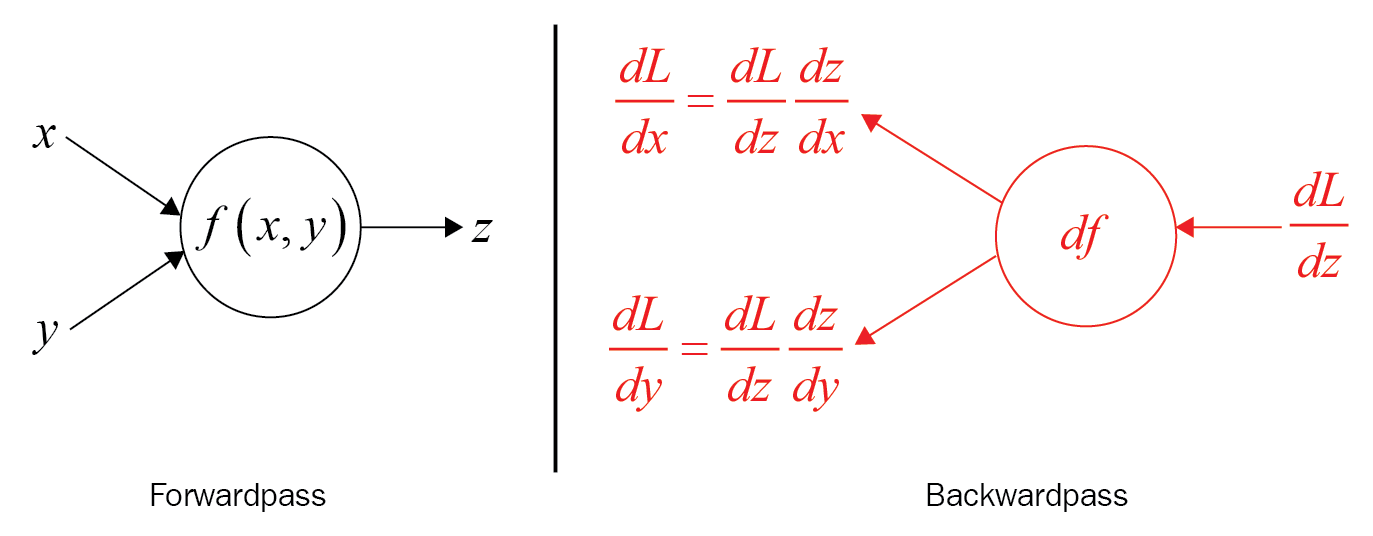## Summary

In this chapter, we laid the foundation of neural networks and walked through the simplest artificial neural network. We learned how to build a single layer neural network using TensorFlow.

We studied the differences in the layers in the Keras model and demonstrated the famous handwritten number recognition with Keras and MNIST.

Finally, we understood what backpropagation is and used the MNIST dataset to build our network and train and test our data.

In the next chapter, we will introduce you to CNNs.

• Mohit Sewak

Mohit Sewak is an Artificial Intelligence scientist with extensive experience and technical leadership in research, architecture, and solutioning of Artificial Intelligence-driven cognitive and automation products and platforms for industries such as IoT, retail, BFSI, and cyber security.

In his current role at QiO Technologies, Mohit leads the reinforcement learning initiative for Industry 4.0 and Smart IoT.

In his previous role, Mohit was associated with IBM Watson Commerce (Software Group) where he led the research/science initiatives for the Watson Cognitive Commerce line of product features and offerings.

Mohit has been the Lead Data Scientist/Analytics Architect for some of the most renowned industry-leading International AI/ DL/ ML software and industry solutions. Mohit is also a thought leader in the field of Artificial Intelligence and Machine Learning and has authored multiple books and scientific publications in this area..

Browse publications by this author
• Md. Rezaul Karim

Md. Rezaul Karim is a researcher, author, and data science enthusiast with a strong computer science background, coupled with 10 years of research and development experience in machine learning, deep learning, and data mining algorithms to solve emerging bioinformatics research problems by making them explainable. He is passionate about applied machine learning, knowledge graphs, and explainable artificial intelligence (XAI). Currently, he is working as a research scientist at Fraunhofer FIT, Germany. He is also a PhD candidate at RWTH Aachen University, Germany. Before joining FIT, he worked as a researcher at the Insight Centre for Data Analytics, Ireland. Previously, he worked as a lead software engineer at Samsung Electronics, Korea.

Browse publications by this author